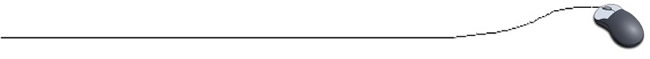Passing Arrays to Methods
(resembles "pass-by-reference")
When discussing arguments/parameters and methods, we talked about passing-by-valueCopies of argument values are sent to the method, where the copy is manipulated and in certain cases, one value may be returned.  While the copied values may change in the method, the original values in main did not change (unless purposely reassigned after the method).

The situation, when working with arrays, is somewhat different.  If we were to make copies of arrays to be sent to methods, we could potentially be copying very large amounts of data.
Not very efficient!

Passing an array mimics a concept called "pass-by-reference", meaning that when an array is passed as an argument, its memory address location (its "reference") is used.  In this way, the contents of an array CAN be changed inside of a method, since we are dealing directly with the actual array and not with a copy of the array.
Note: In Java, a pointer value to the memory address location is used, making arrays "pass-by-value" (and not actually "pass-by-reference" as seen in C++.)

You can pass an entire array, or a single element from an array, to a method.
A method declaration can include array parameters, such as passing the entire array:
public static void testArray(int [ ] num) {
Notice that the int [ ] indicates an array parameter.

A method declaration can include array parameters, such as passing a single array element:
public static void testArray(int number) {
Notice that passing a single array element is similar to passing any single value.
Only the data stored in this single element is passed (not the entire array).

EXAMPLE Snippet:

int [ ] num = {1, 2, 3};
System.out.println("num = " + num + "\n num = " + num + "\n num =" + num);
System.out.println("Now, call the method.");

testArray(num);   // Method call
System.out.println("num = " + num + "\n num = " + num + "\n num =" + num);
. . .

// Method for testing array
// this method will change the original array
public static void testArray(int [ ] value)
{
value = 4;
value = 5;
value = 6;
}

(The values in the array have been changed.
Notice that nothing was "returned".)

 Output: num = 1 num = 2 num = 3 Now, call the method. num = 4 num = 5 num = 6You will need to be careful when sending an array to a method.  Remember that any changes made to the array in the method will change the data in the original array.  Be sure that your intention is to change the original data (thus losing the original data).

Example: Fill an array with 10 integer values.  Pass the array to a method that will add up the values and return the sum.  (In this example, no original data in the array will be altered.  The array information will simply be used to find the sum.)

import java.util.Scanner;

public class FindSum
{
public static void main (String [ ] args)
{
int [ ]  number = new int [ 10];   // instantiate the array
int i;
int sum=0;

for ( i = 0; i < 10; i++ )            // fill the array
{
System.out.println("Enter number: ");
number[ i ] = reply.nextInt( );
}

sum = find_sum(number);   // invoke the method
System.out.println("The sum is " + sum + ".");
}

public static int  find_sum(int [ ] value)  //method to find sum
{
int i, total = 0;
for(i = 0; i < 10; i++)
{
total = total + value[ i ];
}

return (total);
}

}